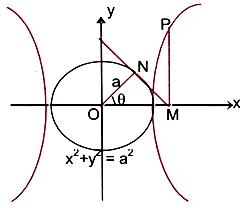# Parametric CoordinatesWe can express the coordinate of a point of the hyperbola x2/a2 – y2/b2 = 1 in terms of a single parameter, say θ.In the adjacent figure OM = a sec θ and PM = b tan θ. Thus any point on the curve, in parametric form is x = a secq, y = b tanθ.

In other words, (a sec θ, b tan θ) is a point on the hyperbola for all values of θ. The point (a secθ, b tanθ) is briefly called the point ‘θ’.

To read more, Buy study materials of Hyperbola comprising study notes, revision notes, video lectures, previous year solved questions etc. Also browse for more study materials on Mathematics here.